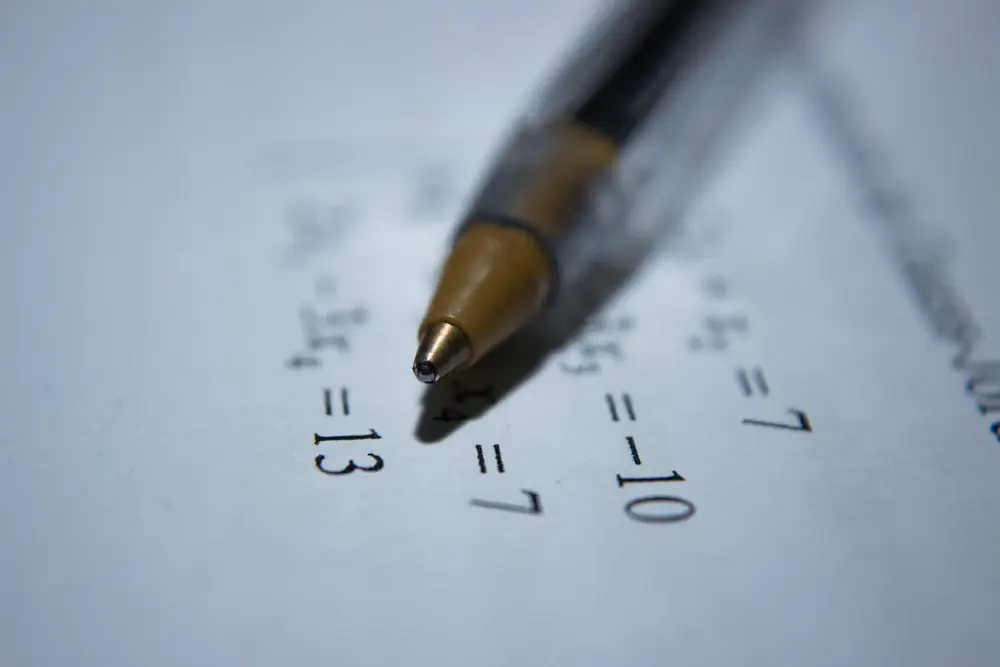# Surds

Surds are numbers left in 'square root form' (or 'cube root form' etc).

a√b + c√b = (a + c)√b
a√b - c√b = (a - c)√b

Examples:
4√7 - 2√7 = 2√7.
5√2 + 8√2 = 13√2

NB1: 5√2 + 3√3 cannot be manipulated because the surds are different (one is √2 and one is √3).
NB2: √a + √b is not the same as √(a + b) .

Multiplication and Division
√ ab = √ a × √ b
√ (a/b) = √ a
√ b

Examples:
√5 × √15 = √ 75
= √25 × √3
= 5√3.

(1 + √3) × (2 - √8)            [The brackets are expanded as usual]
= 2 - √8 + 2√3 - √24
= 2 - 2√2 + 2√3 - 2√6

Rationalising the denominator
It is untidy to have a fraction which has a surd denominator. This can be 'tidied up' by multiplying the top and bottom of the fraction by a surd. This is known as rationalising the denominator, since surds are irrational numbers and so you are changing the denominator from an irrational to a rational number.

Example:
Rationalise the denominator of:
a) 1
√2

b) 1 + 2
1 - √2

a) Multiply the top and bottom of the fraction by √2. The top will become √2 and the bottom will become 2 (√2 times √2 = 2).

b) In situations like this, look at the bottom of the fraction (the denominator) and change the sign (in this case change the plus into minus). Now multiply the top and bottom of the fraction by this.

Therefore:
1 + 2  =   (1 + 2)(1 + √2)  =  1 + √2 + 2 + 22  =  3 + 3√2
1 - √2     (1 - √2)(1 + √2)      1 + √2 - √2 - 2        - 1

= -3(1 + √2)

Algebra

Algebra

Algebra

Algebra

Algebra

Algebra